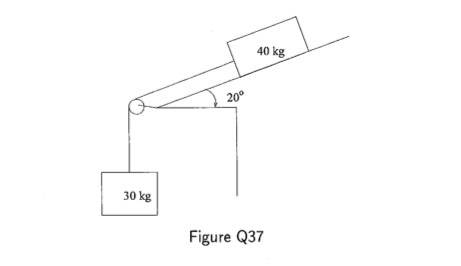# Kinetic Friction + Acceleration

• cd19

## Homework Statement

In the connected crate system the coefficients of dynamic friction between the 40 kg crate and the slope is $$\mu$$d=0.2. There is no friction in the pulley and it can be shown that sliding occurs. Determine the acceleration of the crates## Homework Equations

F = MA
Ff = $$\mu$$d * Nf

## The Attempt at a Solution

I found the friction force of the 40kg crate to be 78.4N, so this is the force required to set the crate in motion.

My attempts from here were to incorporate 'Tension' using the frictional force as applied force and for the 30 kg just simply the weight. From here my efforts have reached a dead end.

## Homework Statement

In the connected crate system the coefficients of dynamic friction between the 40 kg crate and the slope is $$\mu$$d=0.2. There is no friction in the pulley and it can be shown that sliding occurs. Determine the acceleration of the crates## Homework Equations

F = MA
Ff = $$\mu$$d * Nf

## The Attempt at a Solution

I found the friction force of the 40kg crate to be 78.4N, so this is the force required to set the crate in motion.

My attempts from here were to incorporate 'Tension' using the frictional force as applied force and for the 30 kg just simply the weight. From here my efforts have reached a dead end.

How did you get 78.4 N ?

(40)*(9.8)*(.2) = 78.4N ; (Ff = d * Nf)

(40)*(9.8)*(.2) = 78.4N ; (Ff = d * Nf)

The normal force is not mg, it is mg cos(20).

Can you show what you did after that?

ok so the friction force is 73.67N

from here;

a = [F - (40*9.8*.2*cos20) -(30*9.8)/(40 +30)] but I don't know what F is, don't really fully understand the question to be honest is it anything to do with impulse?

Last edited:
Dynamic friction will not tell you how much force is needed to get it moving, that would be static.

So you know that the force retarding the acceleration is from the friction.

Ok so you have found:

the force due to the 30kg mass.
the force due to friction.

What do you think will happen to the 40kg block, even if the 30kg block wasnt attached to it?

(what happens when you put things on a slope and why)

Last edited:
ok so the friction force is 73.67N
OK.
from here;

a = [F - (40*9.8*.2*cos20) -(30*9.8)/(40 +30)] but I don't know what F is
How did you arrive at that equation?

to be honest I'm not entirely sure, seen something like it in an example and tried to use it in this problem. 'f = m(a) ' so I was basically getting the sum of the forces and dividing by the combined mass of the two blocks... am i on the right track?

You are on the right track with F=ma, but if you don't know where you got the equation then getting an answer is pointless.

Do you know how to draw a free body diagram?

yeah but I'm not great at them, any quick tips have an exam tomarrow!.. looking at it now if I find the resultant of the x-y components of the normal force of the 40 kg block then in turn find the resultant of that and the force due to the 30 kg weight and divide by the the mass of the two blocks...

alright anyone tell me if this is correct? ;

(-Ff+M(40)*(g)*sin20 + M(30)(g)= M(70)*a

giving ---> -73.672 +134.072 + 294/ 70 = a

therefore acceleration = 5.06 ms-2

alright anyone tell me if this is correct? ;

(-Ff+M(40)*(g)*sin20 + M(30)(g)= M(70)*a

giving ---> -73.672 +134.072 + 294/ 70 = a

therefore acceleration = 5.06 ms-2
That looks OK.